This preliminary work on adding and multiplying numbers follows on from earlier work with Jim Stone and Alistair Bray on extracting invariances from images. There is a Network paper on this topic which can be retrieved from my publications page.

Two input images are created such that 4x4 patch in left image and 4x4 patch in corresponding region of right image represent two numbers (x and y) in binary format. The binary number is ordered on a row by row basis within the 4x4 patch with bit 0 (lsb) in the top left hand corner and bit 15 (msb) in the bottom right hand corner of the image patch. The sum of these two numbers, z = x+y, varies in a smooth fashion across the surface of the image. z is initially chosen in the range [0,1] and then scaled by 2**16. Given z, we chose x to be a random value between 0 and z, and then we set y = z-x.

A three-layer network was used, with 2x4x4 inputs going to a hidden layer of 3 tanh units and 1 output unit. Half-lives for averaging: U=1, V=500.

The inputs to the network are the following two binary images (no smoothing or normalisation of input patches):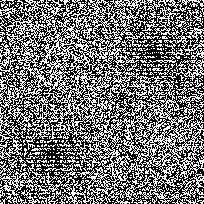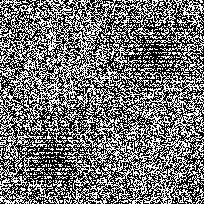The sum of the two patches in each image has an "egg-box" profile (shown on the left). Network performance after 200 epochs is shown on the right.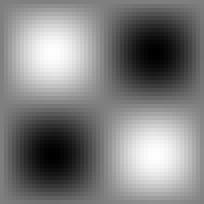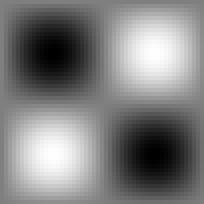## Plot of z versus network output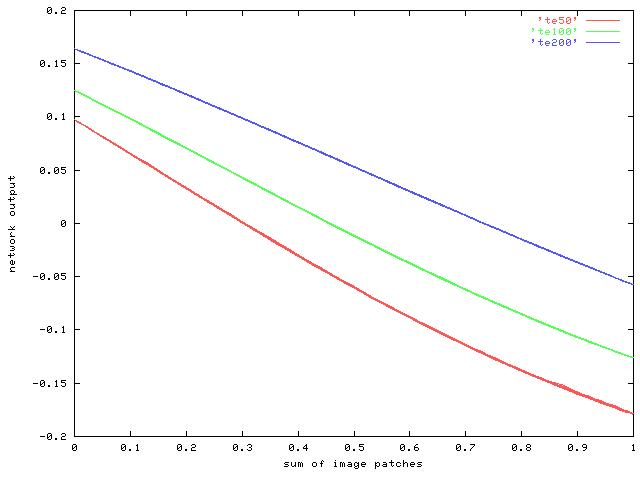[All images here are jpegs which accounts for the poor quality on some of the graphs.]

This plot shows how the network output varies with the desired sum of the input patches after 50 (red), 100 (green) and 200 (blue) iterations. As the line gets straighter during learning, the range of output values is reduced slightly.

## Value of merit function during learning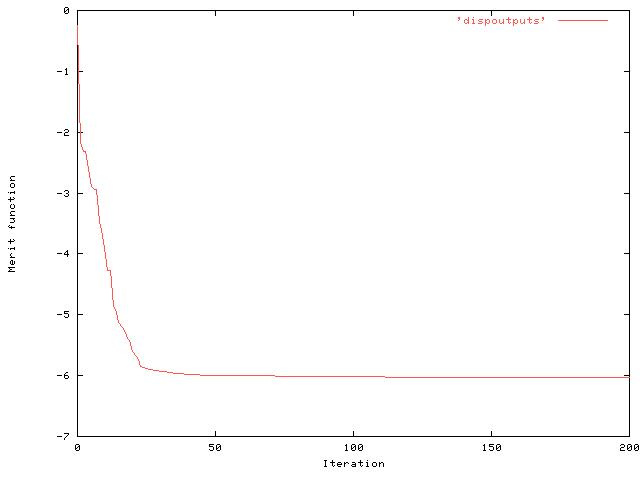Note this is a very low value of the merit function -- typically we used to see values of around 1.8 for the disparity test and around 0.9 for the feature orientation. [It is negative since we are taking logs and the value V/U has gone below 1.0.]

## Correlation between z and network output during learning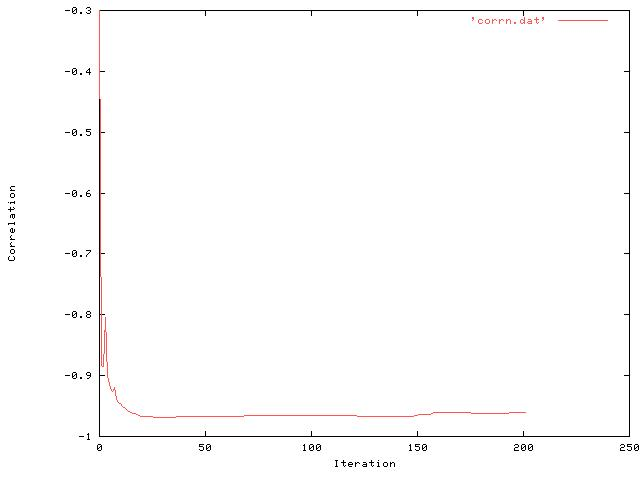Note that the correlation reaches a very high value (-0.97) fairly quickly before decreasing slightly at the end to around (-0.96).

## Testing on unseen data

To test that the network is computing the sum, a new pair of images were created, this time with the sum of the inputs varying in a Gaussian fashion across the two images.

Inputs are just the binary values (no smoothing or normalisation of input patches):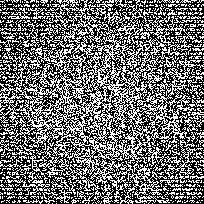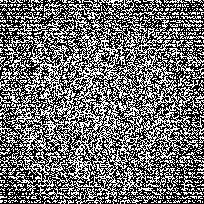The sum of the two patches in each image has a Gaussian profile (shown on the left). Network performance using network trained on egg box data above is shown the right. Correlation between the two is -0.998 (v. good!).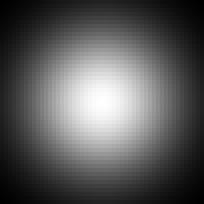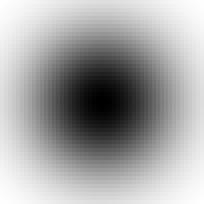## Two layer nets

Seeing how quickly the network learnt, I wondered if the network needed a hidden layer. Using a simple two layer network with 2x4x4 inputs and one tanh output unit I got the following results, varying the range of the short-range half life (r is the correlation between output and desired value).

### Half-life 1. r = -0.34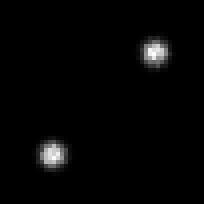### Half-life 3. r = 0.57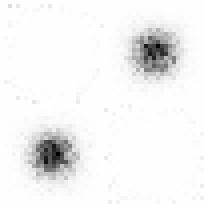### Half-life 5. r = -0.97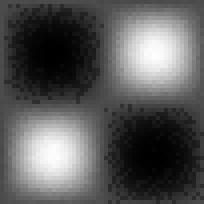In this case, the merit function reached a stable value of -0.8 after around just 10 epochs. When tested on the unseen Gaussian images, correlation was very high (-0.97).

## The weights

The weights in the 2-d network show very clearly what is going on.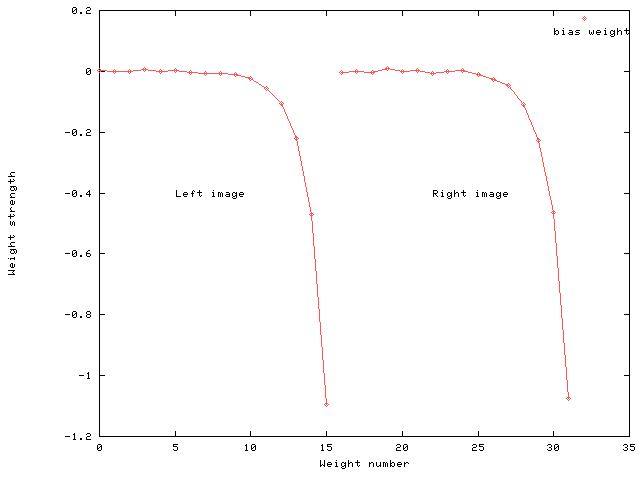Weights 0-15 are the connections from the left image patch to the output unit, and weights 16-31 are the connections from the right image. There is also a bias weight (32). The network has least weight strength for the least significant bit of each number and increasing weight strength for successive bits of each number. To test which weights in the network were important for the task, once the network had been trained on the egg-box data, various weights were set to zero to see how much each weight contributed towards network performance.
 Non-zero weights r 15 -0.667 14-15 -0.912 13-15 -0.963 12-15 -0.971 9-15 -0.969 0-15 -0.968
The above table shows the test correlation for the unseen Gaussian image pair as different weights are set to zero. So, for example the top row of the table shows the correlation when weights 0-14 for both left and right image patches were set to zero, leaving only weight 15. To produce a high correlation of -0.97, the network only needs to keep connections to the 4 most significant bits of each image patch -- the remaining 12 bits of each image patch are effectively redundant.

This can be seen if we test the network with a set of inputs that do not make use of the full range [0..2**16] of possible values of z. (This is using the network trained on the egg-box data with a full-range [0..2**16] of values for z.) The table below shows the correlation for different ranges of z in the test images. So, when the input images only code sums in the range [0..2**8], the correlation between output and desired output is very low (-0.174) and is expected. As the range of possible values for z increases back to [0..2**16], network performance returns to normal.

 [0..2**n] r 8 -0.174 10 -0.466 11 -0.532 12 -0.805 13 -0.944 14 -0.986 15 -0.998 16 -0.978